International
Tables for
Crystallography
Volume D
Physical properties of crystals
Edited by A. Authier

International Tables for Crystallography (2006). Vol. D, ch. 1.1, pp. 13-14

## Section 1.1.4.5.3. Antisymmetric tensors – axial tensors

A. Authiera*

aInstitut de Minéralogie et de la Physique des Milieux Condensés, Bâtiment 7, 140 rue de Lourmel, 75015 Paris, France
Correspondence e-mail: aauthier@wanadoo.fr

| top | pdf |

#### 1.1.4.5.3.1. Tensors of rank 2

| top | pdf |

A bilinear form is said to be antisymmetric if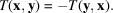Its components satisfy the relations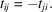The associated matrix, T, is therefore also antisymmetric: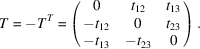The number of independent components is equal to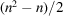, where n is the number of dimensions of the space. It is equal to 3 in a three-dimensional space, and one can consider these components as those of a pseudovector or axial vector. It must never be forgotten that under a change of basis the components of an axial vector transform like those of a tensor of rank 2.

Every tensor can be decomposed into the sum of two tensors, one symmetric and the other one antisymmetric: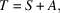with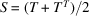and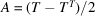.

Example. As shown in Section 1.1.3.7.2, the components of the vector product of two vectors, x and y,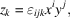are really the independent components of an antisymmetric tensor of rank 2. The magnetic quantities, B, H (Section 1.1.4.3.2), the tensor representing the pyromagnetic effect (Section 1.1.1.3) etc. are axial tensors.

#### 1.1.4.5.3.2. Tensors of higher rank

| top | pdf |

If the rank of the tensor is higher than 2, the tensor may be antisymmetric with respect to the indices of one or several couples of indices.

 (i) Tensors of rank 3 antisymmetric with respect to every couple of indices. A trilinear form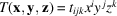is said to be antisymmetric if it satifies the relations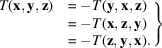Tensor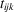has 27 components. It is found that all of them are equal to zero, except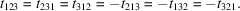The three-times contracted product with the permutations tensor (Section 1.1.3.7.2),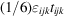, is a pseudoscalar or axial scalar. It is not a usual scalar: the sign of this product changes when one changes the hand of the reference axes, change of basis represented by the matrix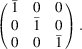Form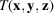can also be written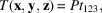where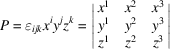is the triple scalar product of the three vectors x, y, z: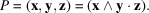It is also a pseudoscalar. The permutation tensor is not a real tensor of rank 3: if the hand of the axes is changed, the sign of P also changes; P is therefore not a trilinear form. Another example of a pseudoscalar is given by the rotatory power of an optically active medium, which is expressed through the relation (see Section 1.6.5.4)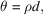where θ is the rotation angle of the light wave, d the distance traversed in the material and ρ is a pseudoscalar: if one takes the mirror image of this medium, the sign of the rotation of the light wave also changes. (ii) Tensor of rank 3 antisymmetric with respect to one couple of indices. Let us consider a trilinear form such that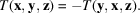Its components satisfy the relation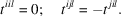The twice contracted product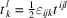is an axial tensor of rank 2 whose components are the independent components of the antisymmetric tensor of rank 3,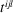.

#### Examples

 (1) Hall constant. The Hall effect is observed in semiconductors. If one takes a semiconductor crystal and applies a magnetic induction B and at the same time imposes a current density j at right angles to it, one observes an electric field E at right angles to the other two fields (see Section 1.8.3.4). The expression for the field can be written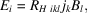where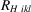is the Hall constant, which is a tensor of rank 3. However, because the direction of the current density is imposed by the physical law (the set of vectors B, j, E constitutes a right-handed frame), one has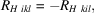which shows thatis an antisymmetric (axial) tensor of rank 3. As can be seen from its physical properties, only the components such that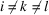are different from zero. These are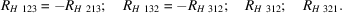(2) Optical rotation. The gyration tensor used to describe the property of optical rotation presented by gyrotropic materials (see Section 1.6.5.4) is an axial tensor of rank 2, which is actually an antisymmetric tensor of rank 3. (3) Acoustic activity. The acoustic gyrotropic tensor describes the rotation of the polarization plane of a transverse acoustic wave propagating along the acoustic axis (see for instance Kumaraswamy & Krishnamurthy, 1980). The elastic constants may be expanded as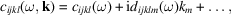where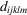is a fifth-rank tensor. Time-reversal invariance requires that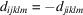, which shows that it is an antisymmetric (axial) tensor.

#### 1.1.4.5.3.3. Properties of axial tensors

| top | pdf |

The two preceding sections have shown examples of axial tensors of ranks 0 (pseudoscalar), 1 (pseudovector) and 2. They have in common that all their components change sign when the sign of the basis is changed, and this can be taken as the definition of an axial tensor. Their components are the components of an antisymmetric tensor of higher rank. It is important to bear in mind that in order to obtain their behaviour in a change of basis, one should first determine the behaviour of the components of this antisymmetric tensor.

### References

Kumaraswamy, K. & Krishnamurthy, N. (1980). The acoustic gyrotropic tensor in crystals. Acta Cryst. A36, 760–762.